Question

What is the slope-intercept equation of the line that is perpendicular to y-4=-2/3(x-6) and that passes through (-2, -2)?

1.thuthao

The slope-intercept equation is: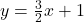Step-by-step explanation:

Given the equation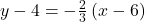comparing it with the point-slope form of the line equation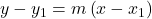where m is the slope

• so the slope of the line is -2/3.

As we know that the slope of the perpendicular line is basically the negative reciprocal of the slope of the line, so

The slope of the perpendicular line will be: 3/2

The point-slope form of the equation of the perpendicular line that goes through (-2, -2) is: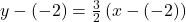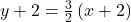writing the line equation in the slope-intercept formsubtract 2 from both sides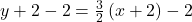Thus, the slope-intercept equation is:Here,

As the slope-intercept form is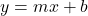where m is the slope and b is the y-intercept

som=3/2

b = y-intercept = 1

Therefore, the slope-intercept equation is: